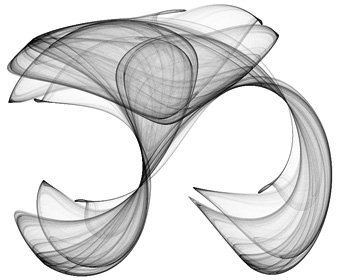## attractorProject contains code to draw attractors. These objects are made with a simple algorithm:
1) take any point p0 = (x0, y0),
2) calculate the next point pn+1 = ν(pn) = (xn+1, yn+1), change the color at pn+1,
3) and repeat step 2 many times...

Formulas to calculate successive pn+1 points are:

xn+1 = f(xn, yn) = sin(t1 * yn) + t3 * cos(t1 * xn)
yn+1 = g(xn, yn) = sin(t2 * xn) + t4 * cos(t2 * yn)

Look at the file attractor_basics.l, you'll find the corresponding code easyli. These formulas are just to start experiments. Other combinations of trigonometric functions will work as well, just be warned: it takes some patience to find interesting ti coefficients.

In the file app.l you will find a complete application to draw attractors. It uses a base `attractor` class to implement all common functions. The derived `custom_attractor` redefines `f` and `g` formulas. There are also some clues how to play with color modifications. Another interesting idea is to change ti's slowly with iterations... try it.

Attractors idea for the project and some coefficient sets based on: Clifford Attractors